# Title 40

## SECTION 1065.660

### 1065.660 THC, NMHC, NMNEHC, CH4, and C2H6 determination.

§ 1065.660 THC, NMHC, NMNEHC, CH4, and C2H6 determination.

(a) THC determination and initial THC/CH4 contamination corrections. (1) If we require you to determine THC emissions, calculate xTHC[THC-FID]cor using the initial THC contamination concentration xTHC[THC-FID]init from § 1065.520 as follows: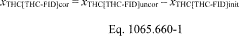Example: xTHCuncor = 150.3 µmol/mol xTHCinit = 1.1 µmol/mol xTHCcor = 150.3 - 1.1 xTHCcor = 149.2 µmol/mol

(2) For the NMHC determination described in paragraph (b) of this section, correct xTHC[THC-FID] for initial THC contamination using Eq. 1065.660-1. You may correct xTHC[NMC-FID] for initial contamination of the CH4 sample train using Eq. 1065.660-1, substituting in CH4 concentrations for THC.

(3) For the NMNEHC determination described in paragraph (c) of this section, correct xTHC[THC-FID] for initial THC contamination using Eq. 1065.660-1. You may correct xTHC[NMC-FID] for initial contamination of the CH4 sample train using Eq. 1065.660-1, substituting in CH4 concentrations for THC.

(4) For the CH4 determination described in paragraph (d) of this section, you may correct xTHC[NMC-FID] for initial THC contamination of the CH4 sample train using Eq. 1065.660-1, substituting in CH4 concentrations for THC.

(b) NMHC determination. Use one of the following to determine NMHC concentration, xNMHC:

(1) If you do not measure CH4, you may omit the calculation of NMHC concentrations and calculate the mass of NMHC as described in § 1065.650(c)(5).

(2) For nonmethane cutters, calculate xNMHC using the nonmethane cutter's penetration fraction (PF) of CH4 and the response factor penetration fraction (RFPF) of C2H6 from § 1065.365, the response factor (RF) of the THC FID to CH4 from § 1065.360, the initial THC contamination and dry-to-wet corrected THC concentration xTHC[THC-FID]cor as determined in paragraph (a) of this section, and the dry-to-wet corrected CH4 concentration xTHC[NMC-FID]cor optionally corrected for initial THC contamination as determined in paragraph (a) of this section.

(i) Use the following equation for penetration fractions determined using an NMC configuration as outlined in § 1065.365(d):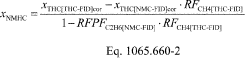Where: xNMHC = concentration of NMHC. xTHC[THC-FID]cor = concentration of THC, initial THC contamination and dry-to-wet corrected, as measured by the THC FID during sampling while bypassing the NMC. xTHC[NMC-FID]cor = concentration of THC, initial THC contamination (optional) and dry-to-wet corrected, as measured by the NMC FID during sampling through the NMC. RFCH4[THC-FID] = response factor of THC FID to CH4, according to § 1065.360(d). RFPFC2H6[NMC-FID] = nonmethane cutter combined ethane response factor and penetration fraction, according to § 1065.365(d). Example: xTHC[THC-FID]cor = 150.3 µmol/mol xTHC[NMC-FID]cor = 20.5 µmol/mol RFPFC2H6[NMC-FID] = 0.019 RFCH4[THC-FID] = 1.05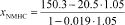xNMHC = 131.4 µmol/mol

(ii) For penetration fractions determined using an NMC configuration as outlined in section § 1065.365(e), use the following equation: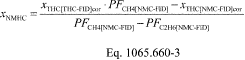Where: xNMHC = concentration of NMHC. xTHC[THC-FID]cor = concentration of THC, initial THC contamination and dry-to-wet corrected, as measured by the THC FID during sampling while bypassing the NMC. PFCH4[NMC-FID] = nonmethane cutter CH4 penetration fraction, according to § 1065.365(e). xTHC[NMC-FID]cor = concentration of THC, initial THC contamination (optional) and dry-to-wet corrected, as measured by the THC FID during sampling through the NMC. PFC2H6[NMC-FID] = nonmethane cutter ethane penetration fraction, according to § 1065.365(e). Example: xTHC[THC-FID]cor = 150.3 µmol/mol PFCH4[NMC-FID] = 0.990 xTHC[NMC-FID]cor = 20.5 µmol/mol PFC2H6[NMC-FID] = 0.020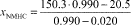xNMHC = 132.3 µmol/mol

(iii) For penetration fractions determined using an NMC configuration as outlined in section § 1065.365(f), use the following equation: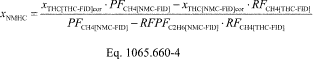Where: xNMHC = concentration of NMHC. xTHC[THC-FID]cor = concentration of THC, initial THC contamination and dry-to-wet corrected, as measured by the THC FID during sampling while bypassing the NMC. PFCH4[NMC-FID] = nonmethane cutter CH4 penetration fraction, according to § 1065.365(f). xTHC[NMC-FID]cor = concentration of THC, initial THC contamination (optional) and dry-to-wet corrected, as measured by the THC FID during sampling through the NMC. RFPFC2H6[NMC-FID] = nonmethane cutter CH4 combined ethane response factor and penetration fraction, according to § 1065.365(f). RFCH4[THC-FID] = response factor of THC FID to CH4, according to § 1065.360(d). Example: xTHC[THC-FID]cor = 150.3 µmol/mol PFCH4[NMC-FID] = 0.990 xTHC[NMC-FID]cor = 20.5 µmol/mol RFPFC2H6[NMC-FID] = 0.019 RFCH4[THC-FID] = 0.980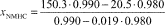xNMHC = 132.5 µmol/mol

(3) For a GC-FID or FTIR, calculate xNMHC using the THC analyzer's response factor (RF) for CH4, from § 1065.360, and the initial THC contamination and dry-to-wet corrected THC concentration xTHC[THC-FID]cor as determined in paragraph (a) of this section as follows: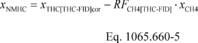Where: xNMHC = concentration of NMHC. xTHC[THC-FID]cor = concentration of THC, initial THC contamination and dry-to-wet corrected, as measured by the THC FID. RFCH4[THC-FID] = response factor of THC-FID to CH4. xCH4 = concentration of CH4, dry-to-wet corrected, as measured by the GC-FID or FTIR. Example: xTHC[THC-FID]cor = 145.6 µmol/mol RFCH4[THC-FID] = 0.970 xCH4 = 18.9 µmol/mol xNMHC = 145.6−0.970 · 18.9 xNMHC = 127.3 µmol/mol

(4) For an FTIR, calculate xNMHC by summing the hydrocarbon species listed in § 1065.266(c) as follows: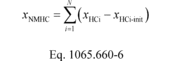Where: xNMHC = concentration of NMHC. xHCi = the C1-equivalent concentration of hydrocarbon species i as measured by the FTIR, not corrected for initial contamination. xHCi-init = the C1-equivalent concentration of the initial system contamination (optional) of hydrocarbon species i, dry-to-wet corrected, as measured by the FTIR. Example: xC2H6 = 4.9 µmol/mol xC2H4 = 0.9 µmol/mol xC2H2 = 0.8 µmol/mol xC3H8 = 0.4 µmol/mol xC3H6 = 0.5 µmol/mol xC4H10 = 0.3 µmol/mol xCH2O = 0.8 µmol/mol xC2H4O = 0.3 µmol/mol xC2H2O2 = 0.1 µmol/mol xCH4O = 0.1 µmol/mol xNMHC = 4.9 + 0.9 + 0.8 + 0.4 + 0.5 + 0.3 + 0.8 + 0.3 + 0.1 + 0.1 xNMHC = 9.1 µmol/mol

(c) NMNEHC determination. Use one of the following methods to determine NMNEHC concentration, xNMNEHC:

(1) If the content of your test fuel contains less than 0.010 mol/mol of ethane, you may omit the calculation of NMNEHC concentrations and calculate the mass of NMNEHC as described in § 1065.650(c)(6).

(2) For a GC-FID or FTIR, calculate xNMNEHC using the THC analyzer's response factors (RF) for CH4 and C2H6, from § 1065.360, and the initial contamination and dry-to-wet corrected THC concentration xTHC[THC-FID]cor as determined in paragraph (a) of this section as follows: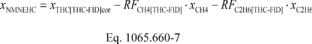Where: xNMNEHC = concentration of NMNEHC. xTHC[THC-FID]cor = concentration of THC, initial THC contamination and dry-to-wet corrected, as measured by the THC FID. RFCH4[THC-FID] = response factor of THC-FID to CH4. xCH4 = concentration of CH4, dry-to-wet corrected, as measured by the GC-FID or FTIR. RFC2H6[THC-FID] = response factor of THC-FID to C2H6. xC2H6 = the C1-equivalent concentration of C2H6, dry-to-wet corrected, as measured by the GC-FID or FTIR. Example: xTHC[THC-FID]cor = 145.6 µmol/mol RFCH4[THC-FID] = 0.970 xCH4 = 18.9 µmol/mol RFC2H6[THC-FID] = 1.02 xC2H6 = 10.6 µmol/mol xNMHC = 145.6 - 0.970 · 18.9 - 1.02 · 10.6 xNMHC = 116.5 µmol/mol

(3) For an FTIR, calculate xNMNEHC by summing the hydrocarbon species listed in § 1065.266(c) as follows: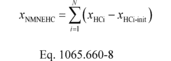Where: xNMNEHC = concentration of NMNEHC. xHCi = the C1-equivalent concentration of hydrocarbon species i as measured by the FTIR, not corrected for initial contamination. xHCi-init = the C1-equivalent concentration of the initial system contamination (optional) of hydrocarbon species i, dry-to-wet corrected, as measured by the FTIR. Example: xC2H4 = 0.9 µmol/mol xC2H2 = 0.8 µmol/mol xC3H8 = 0.4 µmol/mol xC3H6 = 0.5 µmol/mol xC4H10 = 0.3 µmol/mol xCH2O = 0.8 µmol/mol xC2H4O = 0.3 µmol/mol xC2H2O2 = 0.1 µmol/mol xCH4O = 0.1 µmol/mol xNMNEHC = 0.9 + 0.8 + 0.4 + 0.5 + 0.3 + 0.8 + 0.3 + 0.1 + 0.1 xNMNEHC = 4.2 µmol/mol

(d) CH4 determination. Use one of the following methods to determine CH4 concentration, xCH4:

(1) For nonmethane cutters, calculate xCH4 using the nonmethane cutter's penetration fraction (PF) of CH4 and the response factor penetration fraction (RFPF) of C2H6 from § 1065.365, the response factor (RF) of the THC FID to CH4 from § 1065.360, the initial THC contamination and dry-to-wet corrected THC concentration xTHC[THC-FID]cor as determined in paragraph (a) of this section, and the dry-to-wet corrected CH4 concentration xTHC[NMC-FID]cor optionally corrected for initial THC contamination as determined in paragraph (a) of this section.

(i) Use the following equation for penetration fractions determined using an NMC configuration as outlined in § 1065.365(d):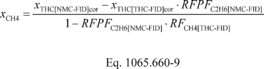Where: xCH4 = concentration of CH4. xTHC[NMC-FID]cor = concentration of THC, initial THC contamination (optional) and dry-to-wet corrected, as measured by the NMC FID during sampling through the NMC. xTHC[THC-FID]cor = concentration of THC, initial THC contamination and dry-to-wet corrected, as measured by the THC FID during sampling while bypassing the NMC. RFPFC2H6[NMC-FID] = the combined ethane response factor and penetration fraction of the nonmethane cutter, according to § 1065.365(d). RFCH4[THC-FID] = response factor of THC FID to CH4, according to § 1065.360(d). Example: xTHC[NMC-FID]cor = 10.4 µmol/mol xTHC[THC-FID]cor = 150.3 µmol/mol RFPFC2H6[NMC-FID] = 0.019 RFCH4[THC-FID] = 1.05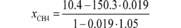xCH4 = 7.69 µmol/mol

(ii) For penetration fractions determined using an NMC configuration as outlined in § 1065.365(e), use the following equation: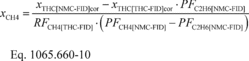Where: xCH4 = concentration of CH4. xTHC[NMC-FID]cor = concentration of THC, initial THC contamination (optional) and dry-to-wet corrected, as measured by the NMC FID during sampling through the NMC. xTHC[THC-FID]cor = concentration of THC, initial THC contamination and dry-to-wet corrected, as measured by the THC FID during sampling while bypassing the NMC. PFC2H6[NMC-FID] = nonmethane cutter ethane penetration fraction, according to § 1065.365(e). RFCH4[THC-FID] = response factor of THC FID to CH4, according to § 1065.360(d). PFCH4[NMC-FID] = nonmethane cutter CH4 penetration fraction, according to § 1065.365(e). Example: xTHC[NMC-FID]cor = 10.4 µmol/mol xTHC[THC-FID]cor = 150.3 µmol/mol PFC2H6[NMC-FID] = 0.020 RFCH4[THC-FID] = 1.05 PFCH4[NMC-FID] = 0.990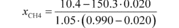xCH4 = 7.25 µmol/mol

(iii) For penetration fractions determined using an NMC configuration as outlined in § 1065.365(f), use the following equation: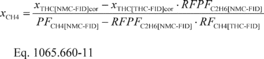Where: xCH4 = concentration of CH4. xTHC[NMC-FID]cor = concentration of THC, initial THC contamination (optional) and dry-to-wet corrected, as measured by the NMC FID during sampling through the NMC. xTHC[THC-FID]cor = concentration of THC, initial THC contamination and dry-to-wet corrected, as measured by the THC FID during sampling while bypassing the NMC. RFPFC2H6[NMC-FID] = the combined ethane response factor and penetration fraction of the nonmethane cutter, according to § 1065.365(f). PFCH4[NMC-FID] = nonmethane cutter CH4 penetration fraction, according to § 1065.365(f). RFCH4[THC-FID] = response factor of THC FID to CH4, according to § 1065.360(d). Example: xTHC[NMC-FID]cor = 10.4 µmol/mol xTHC[THC-FID]cor = 150.3 µmol/mol RFPFC2H6[NMC-FID] = 0.019 PFCH4[NMC-FID] = 0.990 RFCH4[THC-FID] = 1.05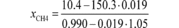xCH4 = 7.78 µmol/mol

(2) For a GC-FID or FTIR, xCH4 is the actual dry-to-wet corrected CH4 concentration as measured by the analyzer.

(e) C2H6 determination. For a GC-FID or FTIR, xC2H6 is the C1-equivalent, dry-to-wet corrected C2H6 concentration as measured by the analyzer.

[76 FR 57462, Sept. 15, 2011, as amended at 81 FR 74184, Oct. 25, 2016]